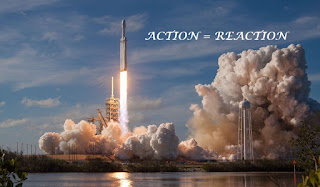### Newton’s Third Law of Motion :

• Newton’s Third Law of Motion states, “When one object exerts a force on a second object, the second one exerts a force on the first that is equal in magnitude and opposite in direction.”
• This law is sometimes called the “Law of Action and Reaction”.
• Even though the forces are equal in magnitude (strength) and opposite in direction, they do not cancel each other.  This law addresses two objects, each with only one force exerted on it.
• Each object is exerting one force on the other object.
• Each object is experiencing only one force.
• The action and reaction forces are reciprocal (opposite) on an object.
• Examples may include:
• A swimmer swimming forward:
• The swimmer pushes against the water (action force), the water pushes back on the swimmer (reaction force) and pushes her forward.
• A ball is thrown against a wall:
• The ball puts a force on the wall (action force), and the wall puts a force on the ball (reaction force) so the ball bounces off.
• A person is diving off a raft:

• The person puts a force on the raft (action force) pushing it, and the raft puts a force on the diver (reaction force) pushing them in the opposite direction.
• A person pushes against a wall (action force), and the wall exerts an equal and opposite force against the person (reaction force).
• The Space Shuttle engines push out hot gases (action force), and the hot gases put a force on the shuttle engines (reaction force) so the shuttle lifts (there is no sling shot doing it!)
•